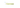# JavaScript Double (==) equals vs Triple (===) equalsIn this tutorial, we are going to learn about the difference between `==` equals and `===` equals in JavaScript with the help of examples.

## Double Equals (==)

If we use double equals `==` to compare the two operands of different types the double equals does the coercion before the comparison.

## What is Coercion?

Coercion means JavaScript tries to convert the operands to the same type before making the comparison.

Let’s see an example.

First, we are comparing the number `1` with the string `"1"` using a double equals operator.

``1 == "1" // true``

In the above example, we will get an output `true` because string `1` is converted into a number `1`.

## Triple Equals (===)

The triple equals (`===`) is used for strict equality checking it means both operands should be same `type` and `value` then only it returns `true` otherwise it returns `false`.

Example:

``1 === "1" // false``

In the above example, we are comparing Number `1` with String `"1"` the value `1` is the same on both sides but the types of both operands are different so that we will get output `false`.

``2 === 2 // true``

Here we are comparing Number `2` with Number `2`, the value and type of both operands are the same so that we will get output `true`.

## Other Examples

``````"hello" === "hello" // true
10 == "10" // true
10 === "10" // false
false == 0 // true
false === 0 //false (boolean false === number 0)``````

## Conclusion

The main difference between tripe and double equals is.

1. Triple equals do the strict comparison in JavaScript it means both value and type should be same to return true.
2. Double equals convert the operands to the same type before making the comparison.

## Top Udemy Courses##### JavaScript - The Complete Guide 2020 (Beginner + Advanced)
45,614 students enrolled
52 hours of video content
View Course##### React - The Complete Guide (incl Hooks, React Router, Redux)
284,472 students enrolled
40 hours of video content
View Course##### Vue - The Complete Guide (w/ Router, Vuex, Composition API)
130,921 students enrolled
48.5 hours of video content
View Course